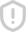### 51单片机红外

#include<reg52.h> void delay(int x) { int i; for (i=x;i>0;i--); } void main () { int ledlx,cycle=400; while(1) { for(ledlx=0;ledlx<cycle;ledlx++) /////////呼吸灯 { P1=0; delay(ledlx); P1=0xff; delay(cycle-ledlx); } for(ledlx=cycle;ledlx>0;ledlx--) { P1=0; delay(ledlx); P1=0xff; delay(cy

...展开详情举报收藏weixin_43491432